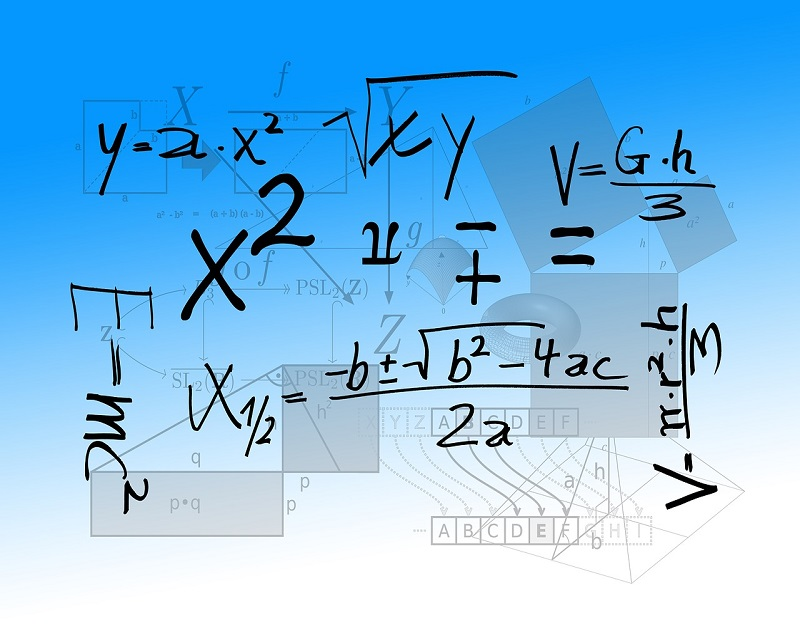# Why Some Students Find Algebra Difficult

Mar 06, 2020Algebraic thinking isn’t just arithmetic with letters standing for numbers. It requires a different kind of thinking.

Many students do find arithmetic difficult to learn, but finally most succeed at least to a certain degree even after lots of practice. What makes it actually possible is that the building blocks of arithmetic-numbers occur naturally around us. Children are aware of a number of applications of arithmetic in the real world starting from counting things, measuring things, purchasing things, dialing a number on a phone, checking scores of a football match and many other similar activities. You might say that these numbers are abstract and in a sense, you are right because you cannot see, feel, hear or smell the number 3. But all these numbers are tied closely to all the concrete things in the very world we live in.

So why is there a problem specifically with algebra? We’ll see to that.

## Students can rarely connect algebra with their everyday life

Algebra is basically a math topic where persons have to think logically about numbers rather than performing computations with numbers.

In algebra, you’re the second step of abstraction (just a figure of speech) away from the everyday world. The Xs and the Ys that you see in the algebra frequently denote numbers in general, not specific numbers.

In algebra, students have to use analytic as well as qualitative reasoning about numbers. But in arithmetic, they have to use numerical and quantitative reasoning with numbers.

Therefore, there’s no doubt that students find it a little difficult to comprehend algebra especially when they shift from primary level to middle-school level mathematics.

## Explanation with an example

You have to implement your algebraic thinking if you want to write a macro for calculating the cells in a spreadsheet (like MS Excel).

The purpose for which the spreadsheet is used doesn’t matter. The spreadsheet can be used for any purpose like calculating scores, keeping a tab on finances, running any business, maintaining a chart of expenses and other similar activities. In such cases, students have to think of numbers rather than thinking directly regarding specific numbers. To be honest, this form of thinking doesn’t come easy.

When students are introduced to algebra, they usually try to solve algebraic problems from the point of view of arithmetic. They tend to apply their arithmetical thinking to solve such sums. This is understandable and is justified because they have just made that transition from primary to middle school, and they are not very acclimatized with algebra. Since they have made an effort in mastering arithmetic in previous classes, it’s expected that they’ll try to apply some of that previous knowledge in this topic. And then the first set of simple algebraic problems even work by that principle. The problem mainly arises when they shift to a fairly advanced algebra.

Take a look at this quadratic equation:

x2 = 2x + 15

We have noticed that many students actually can solve this problem by applying their arithmetical concepts. They tend to guess some numbers substitute them and fine the right answer like in this manner:

x=2,

L.H.S.= 4

R.H.S= 2.2 + 15= 19

Therefore, this is not applicable.

But soon they come up with the right answer when they do x=5.

L.H.S= 25

R.H.S= 5.2+15= 25

Thus, they come up with the answer x=5.

But is this moment feasible enough? What will happen if the answer would have been 267? Can you find that in an instant by applying that method? You would take almost an eternity to get to the right answer.

Thus, to sum up, we can say that the main problem due to which students suffer in algebra is that they tend to solve these sums from the arithmetical point of view. To make this problem into a solution, they must stop thinking arithmetically, and start thinking algebraically. Professional math tutors can help you out in this matter.